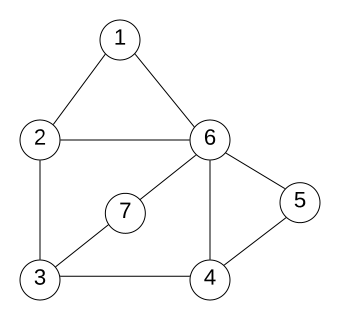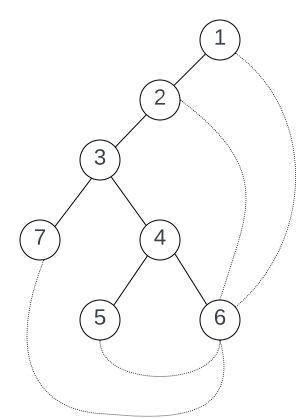# Spanning Tree (Graph Theory)

If $G$ is a connected graph, then the Spanning Tree $T \sube G$ is a minimal connected graph with $V(T) = V(G)$.

The edges $E(G) \setminus E(T)$ are called the chords of $T$ in $G$.

## Example​

$G$ is a connected graph with $|G| = 7$ and $||G|| = 10$.Figure 1 Graph G

Removing edges so that $G$ becomes a minimal connected graph. The chords $E(G) \setminus E(T)$ are depicted as curved lines.Figure 2 Spanning Tree T of G. The curved edges are the chords of T in G.